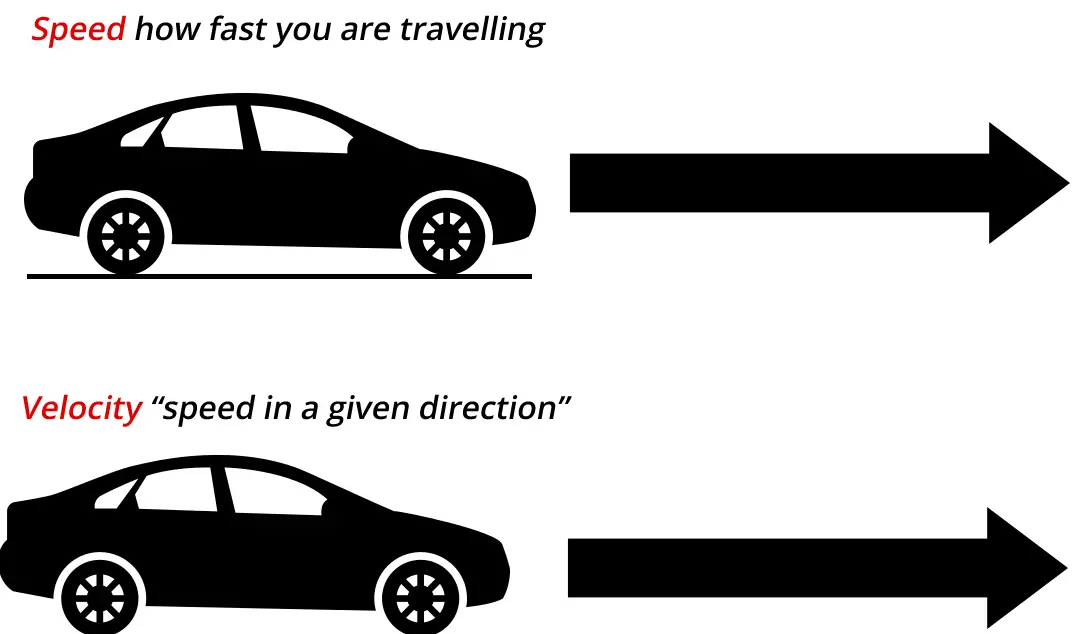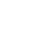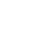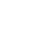# Speed and Velocity Facts

Physics is the science that studies motion and matter and how both work with forces and energy.

It is a really big subject that has a lot of different “branches” of scientific study that include motion, sound, waves, electricity, light, sound, and even astronomy.

This science covers the tiniest particles and atoms all the way to the largest objects in the universe.

Many people get the words speed and velocity confused.

While they can be referenced in what we see and do in our lives, they represent two different physics quantities.

Speed and motion are measured by how fast an object moves relative to a reference point.

### Speed:

When you talk about speed you need to remember that it is the measurement of how fast something is moving as it relates to a point of reference.

It doesn’t have a direction like a vector and is therefore called a “magnitude” or “scalar quantity.”In physics, speed has a formula of Speed = Distance/Time (or speed is equal to distance divided by time) with the abbreviated version of: s=d/t.

Science measures speed using the standard unit of meters per second or m/s. There are two different types of speed measurements:

• Instantaneous speed is the speed of an object at a given moment. An example of this may be a car traveling 40 mph right now, but it may speed up or slow down in the next 30 minutes.
• Average speed is the calculation of the distance that an object has traveled over a specific amount of time. If a car travels 50 miles in an hour, then its average speed is 50 mph. However, during that hour the car may have traveled some of the trip at 60 mph and other parts of the trip at 40 mph. This gives an average speed of 50 mph.

### VelocityVelocity is a bit trickier. It is the rate of change in an object’s position. Velocity has a direction and a magnitude (speed) and is therefore considered to be a vector.

Science uses a formula for the velocity of Velocity = the change in distance/change in time (or Velocity is equal to the change in distance divided by the change in time)

Science uses the same measurement unit of meters per second as they use with speed  = m/s.

In order to calculate what the average velocity of an object is, you need to divide its displacement by how long it took to change position.

### Velocity is a Vector

The difference between speed and velocity is simply this

Speed is how fast an object is moving

Velocity is how fast an object is moving and the direction.

### Example:

Let’s say that you were told that someone you knew was driving at 35 kilometers per hour (35 km/hr) but you didn’t know what direction they were going.

You were only given one value so you couldn’t go to a map and draw an arrow line from any point. In this case, physicists would say that the speed is 35 km/hr by the velocity isn’t known.

However, if you were given the information that your friend is driving at 35 km/hr and going north, then you could have an arrow pointing north with a length of 35. Physicist state that the velocity is 35 km/hr north. You now have two values to work with.

Velocity can be defined as the rate of motion in a specific direction. Average speed is the measure of the distance divided by the time that it took. Velocity can be constant or change with acceleration.

In other words, the speed with a direction is velocity.

### Speed of Light

Scientists have defined the fastest possible speed in the universe as the speed of light, which is 299,792,458 meters per second. Physicists represent almost everything with letters and symbols to make things easier to figure out. The number for the speed of light is represented by the letter “c.”

## Speed and velocity Facts for Kids:

• The speedometer in a car is an example of instantaneous speed.
• The Earth has an intense gravitational pull. In order to escape the Earth, velocity is the speed needed to overcome gravity. This is 25,000 miles per hour.
• Another way of writing the speed of light is 186,282 miles per second.
• Dry air has less resistance due to the lack of moisture. The speed of sound in dry air travels at 343.2 meters per second.
• Galileo used mathematics in his discoveries in astronomy and is given credit for being the first scientist to measure speed as distance over time.

### Q&A:

What is the definition of speed?
the measurement of how fast something is moving as it relates to a point of reference

What is the definition of velocity?
the rate of motion in a specific direction

Who is given credit for discovering the measurement of speed as distance over time?
Galileo

What is the fastest speed in the universe?
speed of light

What are the two types of speed?
instantaneous and average

Is an object’s velocity always the same?
no

•History
•Science
•Geography
•Biography
•US History